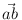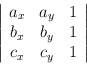Next: Real Segments ( real_segment Up: Basic Data Types for Previous: Iso-oriented Rational Rectangles (   Contents   Index

# Real Points ( real_point )

Definition

An instance of the data type real_point is a point in the two-dimensional plane R2. We use (x, y) to denote a real point with first (or x-) coordinate x and second (or y-) coordinate y.

#include < LEDA/geo/real_point.h >

Types

 real_point::coord_type the coordinate type (real). real_point::point_type the point type (real_point). real_point::float_type the corresponding floating-point type (point).

Creation

 real_point p introduces a variable p of type real_point initialized to the point (0, 0). real_point p(real x, real y) introduces a variable p of type real_point initialized to the point (x, y). real_point p(const point& p1, int prec = 0) introduces a variable p of type real_point initialized to the point p1. (The second argument is for compatibility with rat_point.) real_point p(const rat_point& p1) introduces a variable p of type real_point initialized to the point p1. real_point p(double x, double y) introduces a variable p of type real_point initialized to the real point (x, y).

Operations

 real p.xcoord() returns the first coordinate of p. real p.ycoord() returns the second coordinate of p. int p.orientation(const real_point& q, const real_point& r) returns orientation(p, q, r) (see below). real p.area(const real_point& q, const real_point& r) returns area(p, q, r) (see below). real p.sqr_dist(const real_point& q) returns the square of the Euclidean distance between p and q. int p.cmp_dist(const real_point& q, const real_point& r) returns compare(p.sqrdist(q), p.sqrdist(r)). real p.xdist(const real_point& q) returns the horizontal distance between p and q. real p.ydist(const real_point& q) returns the vertical distance between p and q. real p.distance(const real_point& q) returns the Euclidean distance between p and q. real p.distance() returns the Euclidean distance between p and (0, 0). real_point p.translate(real dx, real dy) returns p translated by vector (dx, dy). real_point p.translate(double dx, double dy) returns p translated by vector (dx, dy). real_point p.translate(const real_vector& v) returns p+ v, i.e., p translated by vector v. Precondition v.dim() = 2. real_point p + const real_vector& v returns p translated by vector v. real_point p - const real_vector& v returns p translated by vector - v. real_point p.rotate90(const real_point& q, int i=1) returns p rotated about q by an angle of i x 90 degrees. If i > 0 the rotation is counter-clockwise otherwise it is clockwise. real_point p.rotate90(int i=1) returns p.rotate90( realpoint(0, 0), i). real_point p.reflect(const real_point& q, const real_point& r) returns p reflected across the straight line passing through q and r. real_point p.reflect(const real_point& q) returns p reflected across point q. real_vector p - const real_point& q returns the difference vector of the coordinates.

Non-Member Functions

 int cmp_distances(const real_point& p1, const real_point& p2, const real_point& p3, const real_point& p4) compares the distances (p1,p2) and (p3,p4). Returns +1 (-1) if distance (p1,p2) is larger (smaller) than distance (p3,p4), otherwise 0. real_point center(const real_point& a, const real_point& b) returns the center of a and b, i.e. a +/2. real_point midpoint(const real_point& a, const real_point& b) returns the center of a and b. int orientation(const real_point& a, const real_point& b, const real_point& c) computes the orientation of points a, b, and c as the sign of the determinanti.e., it returns +1 if point c lies left of the directed line through a and b, 0 if a,b, and c are collinear, and -1 otherwise. int cmp_signed_dist(const real_point& a, const real_point& b, const real_point& c, const real_point& d) compares (signed) distances of c and d to the straight line passing through a and b (directed from a to b). Returns +1 (-1) if c has larger (smaller) distance than d and 0 if distances are equal. real area(const real_point& a, const real_point& b, const real_point& c) computes the signed area of the triangle determined by a,b,c, positive if orientation(a, b, c) > 0 and negative otherwise. bool collinear(const real_point& a, const real_point& b, const real_point& c) returns true if points a, b, c are collinear, i.e., orientation(a, b, c) = 0, and false otherwise. bool right_turn(const real_point& a, const real_point& b, const real_point& c) returns true if points a, b, c form a righ turn, i.e., orientation(a, b, c) < 0, and false otherwise. bool left_turn(const real_point& a, const real_point& b, const real_point& c) returns true if points a, b, c form a left turn, i.e., orientation(a, b, c) > 0, and false otherwise. int side_of_halfspace(const real_point& a, const real_point& b, const real_point& c) returns the sign of the scalar product (b - a)*(c - a). If ba this amounts to: Let h be the open halfspace orthogonal to the vector b - a, containing b, and having a in its boundary. Returns +1 if c is contained in h, returns 0 is c lies on the the boundary of h, and returns -1 is c is contained in the interior of the complement of h. int side_of_circle(const real_point& a, const real_point& b, const real_point& c, const real_point& d) returns +1 if point d lies left of the directed circle through points a, b, and c, 0 if a,b,c,and d are cocircular, and -1 otherwise. bool inside_circle(const real_point& a, const real_point& b, const real_point& c, const real_point& d) returns true if point d lies in the interior of the circle through points a, b, and c, and false otherwise. bool outside_circle(const real_point& a, const real_point& b, const real_point& c, const real_point& d) returns true if point d lies outside of the circle through points a, b, and c, and false otherwise. bool on_circle(const real_point& a, const real_point& b, const real_point& c, const real_point& d) returns true if points a, b, c, and d are cocircular. bool cocircular(const real_point& a, const real_point& b, const real_point& c, const real_point& d) returns true if points a, b, c, and d are cocircular. int compare_by_angle(const real_point& a, const real_point& b, const real_point& c, const real_point& d) compares vectors b-a and d-c by angle (more efficient than calling compare_by_angle(b-a,d-x) on vectors). bool affinely_independent(const array& A) decides whether the points in A are affinely independent. bool contained_in_simplex(const array& A, const real_point& p) determines whether p is contained in the simplex spanned by the points in A. A may consist of up to 3 points. Precondition The points in A are affinely independent. bool contained_in_affine_hull(const array& A, const real_point& p) determines whether p is contained in the affine hull of the points in A.Next: Real Segments ( real_segment Up: Basic Data Types for Previous: Iso-oriented Rational Rectangles (   Contents   Index